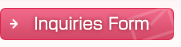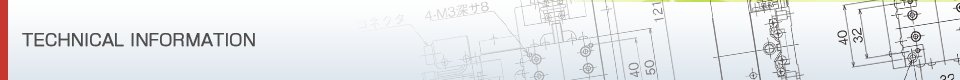## Measuring Instruments & Others

### Laser Auto Collimator

#### Measurement principle of laser auto collimator

Figure 1 shows the optical system. The beam that exits from the semiconductor laser will be focused onto the pinhole (P) with the aid of collecting lens (L1).

Although the laser beam is polarized light beam P horizontal to the paper surface, it spreads after exiting from the pinhole (P) first then penetrates the polarizing beam splitter (PBS), passes through the 1/4 wavelength plate and becomes a circularly polarized light from a linearly polarized light. Then, this beam will be emitted as a parallel beam by means of the collimator lens (L2) via the mirrors (M1 to M4).

This parallel beam will be reflected by the mirror for measurement (M5) and become a circularly polarized light in a reverse rotation direction. The beam will enter the 1/4 wavelength plate via the mirrors (M1 to M4) once again. At this stage, the circularly polarized light in a reverse rotation direction becomes the polarized light beam S which is perpendicular to the paper surface, then it will be reflected by the polarizing beam splitter (PBS) and enter the position detection sensor (CCD).

What is shown in Figure 2 is the light path excluding the mirrors (M1 to M4) shown in Figure 1.When the mirror for measurement (M5) is inclined only by θ, the beam which is reflected by M5 and directed back toward its source enters the collimator lens (L2) under the condition that it remains inclined only by 2 θ and produces an image of the pinhole (P) at a position which is deviated only by d from the center line on PSD. This has such a relation as d = ftan2θ≒ 2fθ.

For this reason, detecting d enables the tilt angle θ of mirror for measurement (M5) to be found.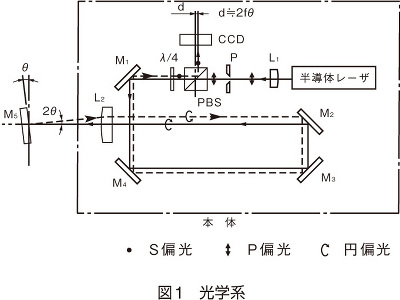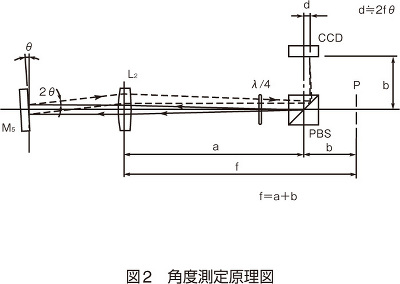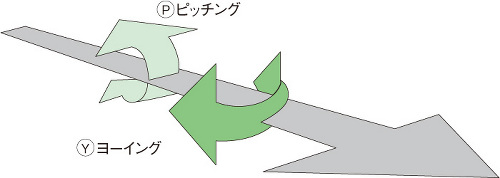#### Example of use of LAC-S

##### Straightness of guide face
The plane mirror slides a pedestal which is fixed in position along the guide face, then the amount of travel of cross-hair image which is measured by the plane mirror will be read.##### Straightness measurement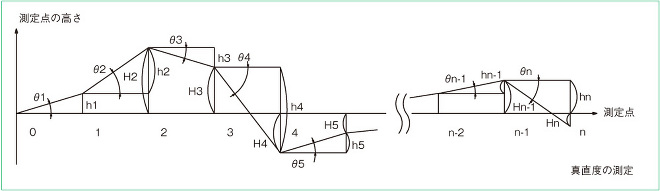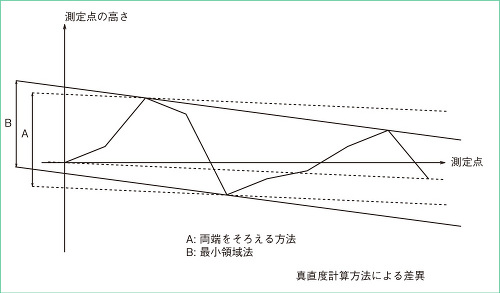In the straightness measurement, h and H of each point are measured based on θ at each measurement point according to the two-point chain method, as shown in the Figure.
There are two measurement methods. One of which is a general "method (A) by which H is calibrated while making heights at both sides uniform and a difference between max. and min. values serves as a straightness".
The other is the "minimum zone method (B)" of JIS that defines that "A height at one end remains zero ... (snip) ... Measure a difference in height between both straight lines when a gap between two parallel straight lines to each other between which a diagrammatic drawing which is drawn after measuring a height of each point is reduced to a minimum".
##### Measurement of rotation angle of rotary table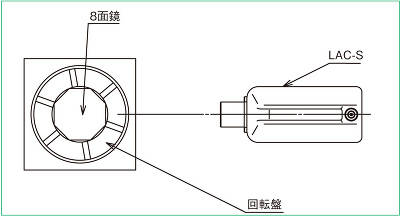A multifaceted mirror is used to measure an accuracy of division of the rotary table or indexing table.
Because 360 degrees are divided into 8 equal parts in a 8-plane mirror, the mirror can act as a basis for indexing an accurate angle in 45 degrees.
Also, using a 12-plane mirror enables measurement to be made in 30 degrees.
##### Deflection of resilient pieceA tiny amount of change can be found by securing a reflecting mirror to a resilient piece and making observations with LAC-S.